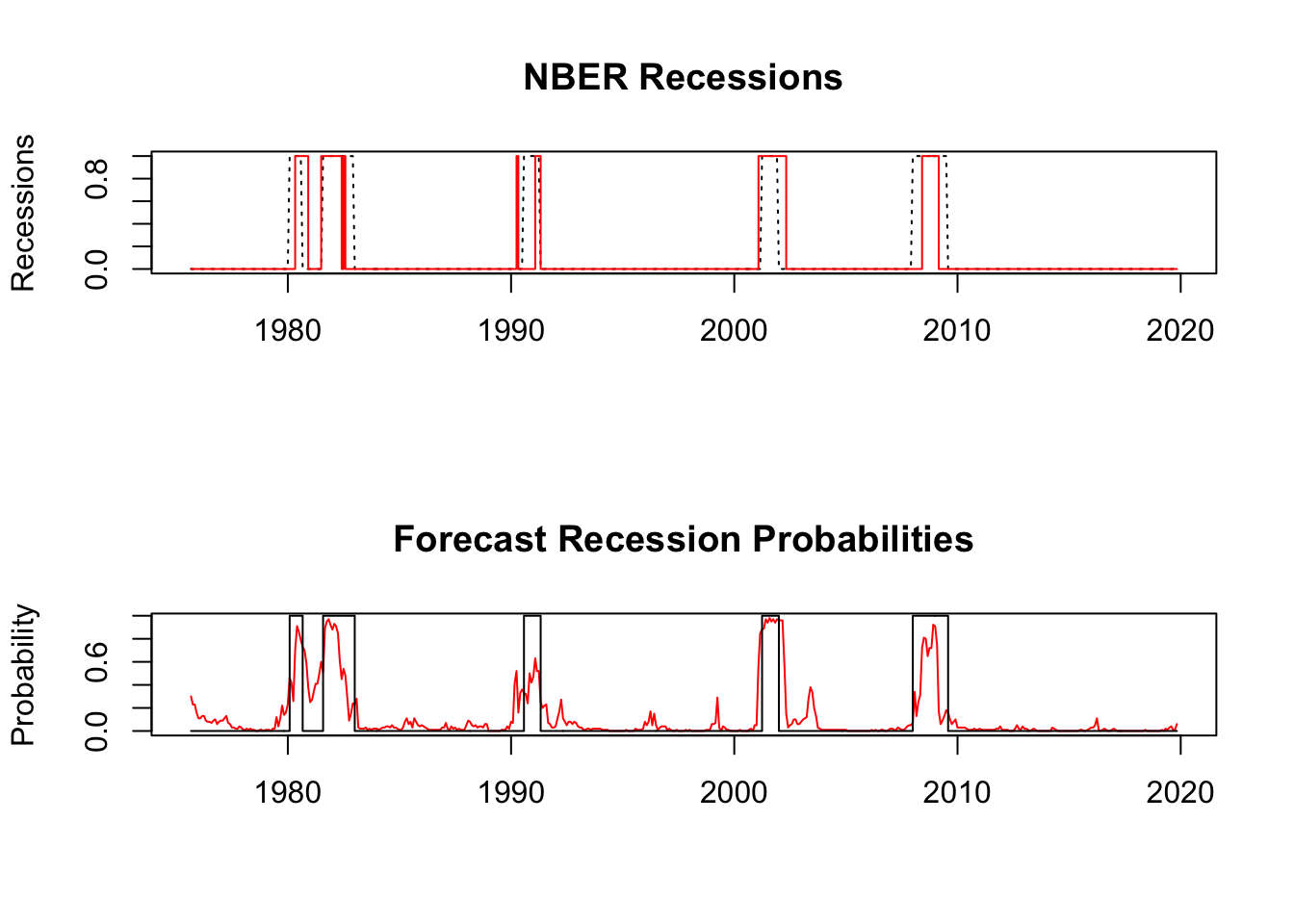### Model Error Rate

We discuss the model details below, but based on training data going back to 1958, the model error rate is 6.78%. The error rate is defined as the number of months where the forecast was incorrect divided by the total number of months in the sample.

### Plot of the results

The model is currently forecasting NO RECESSION. The probability of a recession next month is currently 6%.### Investment Strategy

The backtest measures the accuracy of the SVM predictions versus the actual recession markers as determined by the NBER.

From an investment strategy standpoint, we go long the S&P 500 when a recession is not forecasted and switch to cash when a recession is forecasted. This provides a modest outperformance versus a buy and hold strategy, as shown in the table below.

strategy_ret actual_ret ret
Annualized Return 0.09 0.10 0.08
Annualized Std Dev 0.14 0.13 0.15
Annualized Sharpe (Rf=0%) 0.69 0.76 0.55
StdDev Sharpe (Rf=0%, p=95%): 0.21 0.23 0.17
VaR Sharpe (Rf=0%, p=95%): 0.14 0.15 0.11
ES Sharpe (Rf=0%, p=95%): 0.08 0.09 0.07
Worst Drawdown 0.36 0.31 0.53
Semi-Deviation 0.03 0.03 0.03

strategy_ret is the return from following our forecast, actual_ret is the (theoretical) return one could achieve investing with the NBER decision. The returns in the actual_ret are theoretical because they are not actually achievable since the NBER does not declare recessions until many months after the recession has started, but it shows us the best case scenario. The last column, ret, shows the buy and hold return of the S&P 500.

This strategy increases the Sharpe ratio from 0.551 to 0.692, which is pretty close to the theoretical max of 0.759.

### Code, data, and sources

The full R code is available on Github.

The sample data will automatically load and be stored in the object dat. Here are the important variables.

fwdstate is 1 if the next month is in a recession and -1 otherwise. The model is fwdstate ~ payems + sp500 + yld_curve + man_prod where each of the four independant variables includes the current value plus the most recent 11 lagged months, for a total of 48 variables.

Importantly, there is a lag of 12 months on the data used to forecast recession (the fwdstate variable), so that there is no look ahead bias.